# How to import an excel file into Python using Pandas?

• Last Updated : 17 Aug, 2020

It is not always possible to get the dataset in CSV format. So, Pandas provides us the functions to convert datasets in other formats to the Data frame. An excel file has a ‘.xlsx’ format.

Before we get started,  we need to install a few libraries.

Attention geek! Strengthen your foundations with the Python Programming Foundation Course and learn the basics.

To begin with, your interview preparations Enhance your Data Structures concepts with the Python DS Course. And to begin with your Machine Learning Journey, join the Machine Learning - Basic Level Course

```pip install pandas
pip install xlrd
```

For importing an Excel file into Python using Pandas we have to use pandas.read_excel() function.

Return: DataFrame or dict of DataFrames.

Let’s suppose the Excel file looks like this: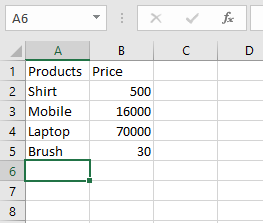Now, we can dive into the code.

Example 1: Read an Excel file.

## Python3

 `import` `pandas as pd`` ` `df ``=` `pd.read_excel(``"sample.xlsx"``)``print``(df)`

Output: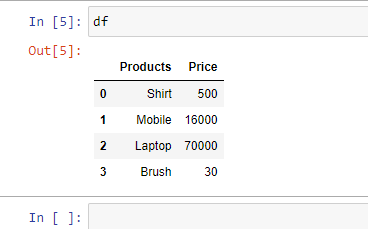Example 2: To select a particular column, we can pass a parameter “index_col“.

## Python3

 `import` `pandas as pd`` ` `# Here 0th column will be extracted``df ``=` `pd.read_excel(``"sample.xlsx"``,``                   ``index_col ``=` `0``)  `` ` `print``(df)`

Output: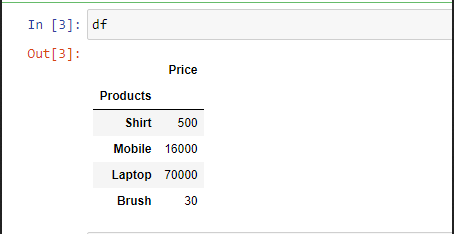Example 3: In case you don’t prefer the initial heading of the columns, you can change it to indexes using the parameter “header”.

## Python3

 `import` `pandas as pd`` ` `df ``=` `pd.read_excel(``'sample.xlsx'``,``                   ``header ``=` `None``)``print``(df)`

Output: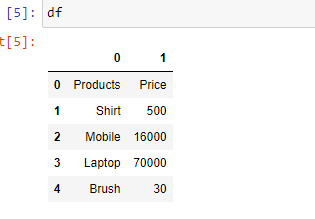Example 4: If you want to change the data type of a particular column you can do it using the parameter “dtype“.

## Python3

 `import` `pandas as pd`` ` `df ``=` `pd.read_excel(``'sample.xlsx'``, ``                   ``dtype ``=` `{``"Products"``: ``str``,``                            ``"Price"``:``float``})``print``(df)`

Output: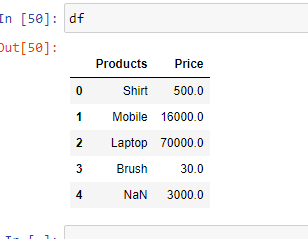Example 5: In case you have unknown values, then you can handle it using the parameter “na_values“. It will convert the mentioned unknown values into “NaN”

## Python3

 `import` `pandas as pd``df ``=` `pd.read_excel(``'sample.xlsx'``, ``                   ``na_values ``=``[``'item1'``, ``                               ``'item2'``])``print``(df)`

Output: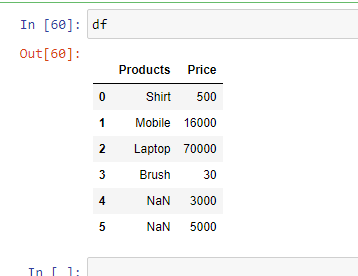My Personal Notes arrow_drop_up